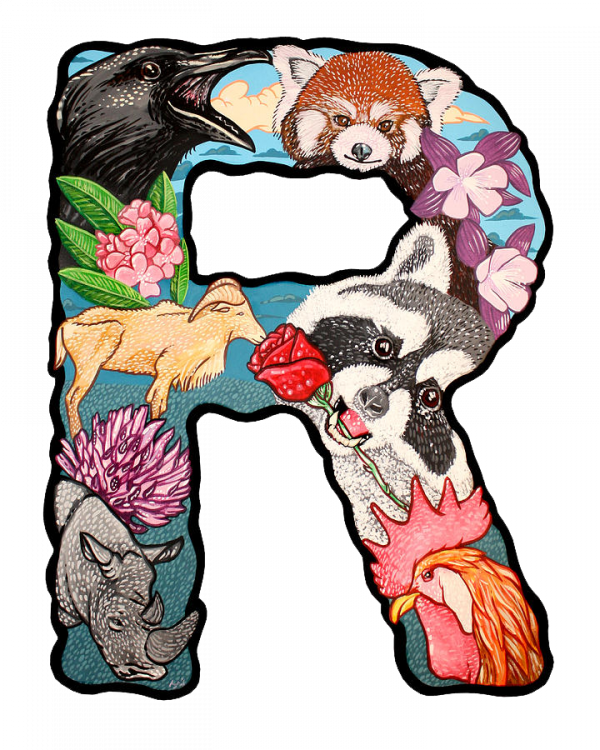With many years of do scientific computing works by VB.NET language, I'm curious if there's a way to scripting my VB.NET library. After learn R language in my school college study, i wondering if i can combine the R language its vectorized programming feature with my VB.NET library natively. So, this idea bring the R# language.

The R# language is born in the idea of bring the vectorization programming language feature on the .NET platform. there are some vectorization programming language like MATLAB language, S language and R language, all of them is exists as the language prototype candidates for design my new language. after the language feature study and do some background investigation works, the R language was choosen as the new vectorization programming language prototype on .NET platform, so this new vectorization programming language is named R# as this new language it is a kind of dialect language which is derive from the R language.

here are some resource links that may be useful for learn R/R# language if you are interesting in R# language:

## Design of the R# Interpreter

How it works?

The R# language is a kind of interpreted programming language currently, and its interpreter is consists with 4 modules: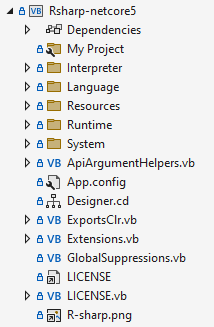• Interpreter: contains the R# language interpreter source code, all of the expression class model definition.
• Language: contains the necessary code for parse the language tokens from the input script text and the syntax parser for create the corresponding R# expression object based on the language token sequence and the language context.
• Runtime: contains the necessary code for imports the external .NET function and the runtime environment definition for run the R# expression evaluation. this folder also contains some primitive R# function for manipulate your dataset, example as lapply, sapply, list, which, etc.
• System: contains the code for the runtime configuration and the third part package loader and tools for build your own R# package.

By combining the code in these 4 modules, we can create a workflow to run the R# script, interop R# script with the existed function in our .NET library and evaluate the R# expression to produce .NET object.

### Workflow: Run R# code

Here is a workflow figure that can be used for illustrate how to run the R# code input: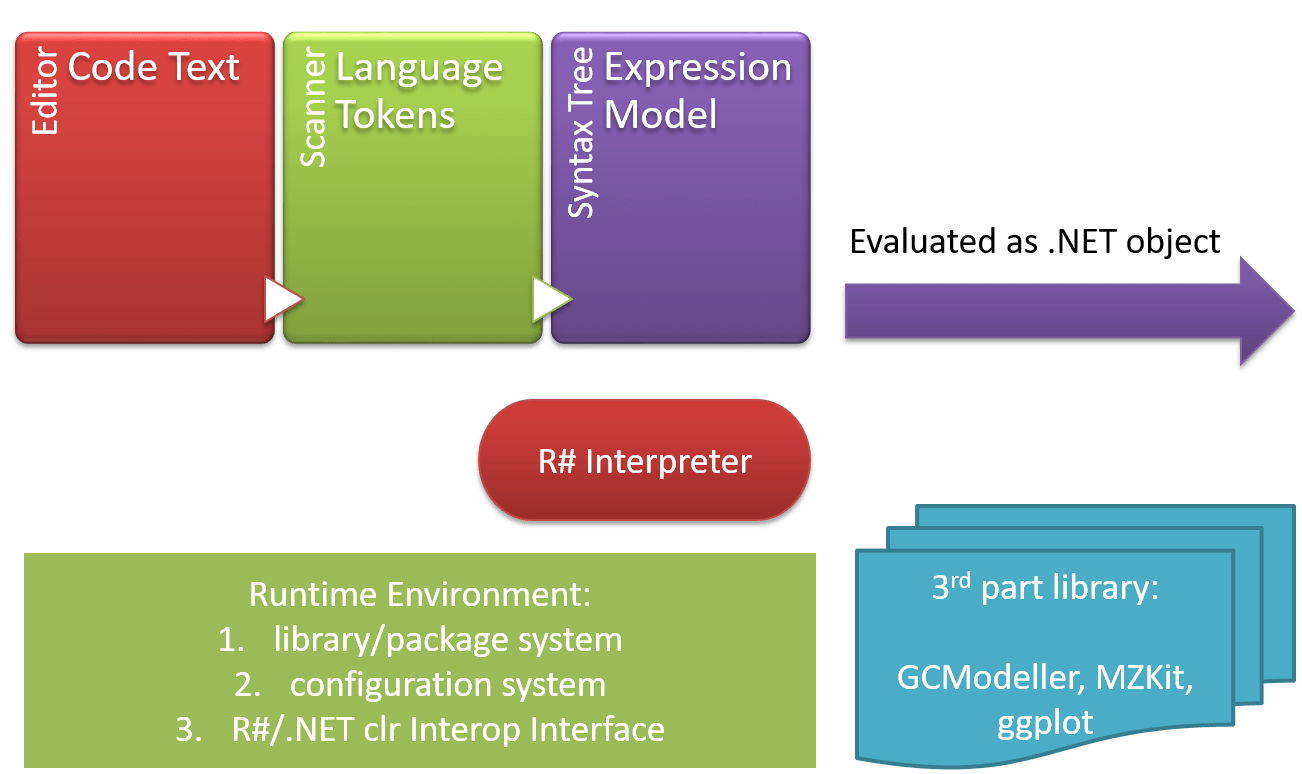1. R# environment initialization: at the very begining of the R# system initialization, the code modules of the R# system will be called for: a) load configuration file, b) initialize the global environment, c) hook the all of the .NET api function which is inside of the R# base package, d) then load startup packages and initialize of the runtime environment. Finally the R# is ready for run our script code.
2. The input script text then will be parsed as the R# language tokens by the scanner object which is defined in the language namespace. the language token sequence is output from the scanner its char walking operation. the order of the language tokens in the generated token sequence is the syntax context information for create the syntax tree by the syntax analysis module in R# interpreter. and after build the syntax tree model from the token sequence, the script text is parsed as a R# program: a collection of the expression models.
3. the expression model of R# language is the very fundamental model for produce result value based on a given evaluation context, so we can abstract the R# expression model as a base class object:
Namespace Interpreter.ExecuteEngine

''' <summary>
''' An expression object model in R# language interpreter
''' </summary>
Public MustInherit Class Expression

''' <summary>
''' Evaluate the R# expression for get its runtime value result.
''' </summary>
''' <param name="envir"></param>
''' <returns></returns>
Public MustOverride Function Evaluate(envir As Environment) As Object

End Class
End Namespace

### Code Demo in VisualBasic

The R# language interpreter is written in VB.NET language originally, so the R# language is fully compatible with the .NET runtime. which means you can embeding the R# environment into your .NET application, this will gives the ability to scripting your .NET library. Here is a full example code about run a R# script file in a VB.NET application on github: "RunRScriptFile".

first we should have a runtime configuration file for run the initialization workflow for the R# language interpreter runtime. the runtime configuration file is a xml file and it can be generated automatically if it is missing from the given file location:

Dim R As RInterpreter = RInterpreter.FromEnvironmentConfiguration(
configs:="/path/to/config.xml"
)

if some external 3rd part R# library dll file is not located in the application directory or library folder, then you should set the dll directory folder path via config of the runtime by:

If Not SetDllDirectory.StringEmpty Then
Call R.globalEnvir.options.setOption("SetDllDirectory", SetDllDirectory)
End If

Load some startup packages before run the given R# script file:

' Call R.LoadLibrary("base")
packageName:=pkgName,
silent:=silent,
ignoreMissingStartupPackages:=ignoreMissingStartupPackages
)
Next

Finally, we can run the script code via the Source function which is exported from the R# interpreter:

result = R.Source(filepath)

if you just want to evaluate the script text, not expected run code from a text file, then you can try the Evaluate function which is exported from the R# interpreter engine:

' Run script by invoke method
Call R.Evaluate("
# test script
let word as string = ['world', 'R# user', 'GCModeller user'];
let echo as function(words) {
print( Hello ${ words }! ); } echo(word); ") ## Comparison between R# and LINQ As we mention above, the R# language is a kind of the vectorization programming language. So a lot of operation in R# programming is vectorized, which means we can do many times of the same operation in just one expression. Although the LINQ language features in .NET platform provides some vectorization programming liked language feature for all .NET language, but it is still a bit of inconvenient when compares with the R/R# language, here is are some examples: ### 1. arithmetic Here we can do some simple math like addition, subtraction, multiplication and division via LINQ: {1,2,3,4,5}.Select(Function(xi) xi + 5).ToArray and do the exact same math operation in R# language will be more simple: [1, 2, 3, 4, 5] + 5; #  6 7 8 9 10 Here is the operators that supported in the R# environment: operator description example compares VB + addition a + b a + b - subtraction a - b a - b * multiplication a * b a * b / division a / b a / b \ integer division a \ b a \ b % mod a % b a Mod b ! not !a Not a == equals a == b a = b != not equals a != b a <> b && and a && b a AndAlso b || or a || b a OrElse b like string pattern matched a like$"\d+" a Like "*.jpg"
in contains a in b b.ContainsKey(a)

### 2. Math function

Using the math function is also super elegant and simple when the R# language is compares with the .NET LINQ:

log10([10, 100, 1000, 10000, 100000]);

.NET LINQ:

{10, 100, 1000, 10000, 100000}.Select(AddressOf Math.Log10).ToArray()

### 3. LINQ function

Although most of the R# script code can be Vectorized, but when we deal with a collection of complex composed dataset in R# script, some loop liked operation is still needed. Although there is the for loop or while loop in R# language, but these loop code in R# programming is not recommended in most of time. Like the original R language, the apply family function can be used for such purpose.

sapply or lapply function in R# language is a kind of LINQ liked function that could be used for the purpose of deal with the complex data collection.

• sapply means sequence apply, which can be Equivalent to the Select function in LINQ. the sapply function accept collection data in R# language and then produce a new vector data.
• lapply means list apply, which can be Equivalent to the ToDictionary function in LINQ. the lapply function is working as the sapply function, accept collection data in R# language but produce a new named key-value paired list data.

Here is an example about the usage of sapply and lapply function in R# language and the corresponding comparison code in LINQ:

[1,2,3,4,5] |> sapply(xi -> xi + 5);
#      6  7  8  9  10
{1,2,3,4,5}.Select(Function(xi) xi + 5).ToArray()

Then, if your want to filter out some un-wanted data in your input data collection, you can apply of the Where function in .NET LINQ. And as the same as the LINQ it does, the R# language is also have a data filter in a data processing pipeline. The LINQ function Where conditional filter is equivalent to the R# function named which, here is an example:

' filter data in .NET LINQ by Where
{1,2,3,4,5}
.Where(Function(x) x > 3)
.ToArray()
# filter data in R# language by which
[1,2,3,4,5]
|> which(x -> x > 3)
;

# another conditional filter syntax in original R language style
x = [1,2,3,4,5];
x[which(x > 3)];
# more simple way:
x[x > 3];

## Comparison between R# and VisualBasic

besides the Vectorization programming feature in R# language is the biggest difference when compares with the VisualBasic.NET language, there are a lot of other language feature that can distinct the R# language and the VisualBasic.NET language.

### 1. declare new function

The function is the basic module in our program, we can build a complex application by the combination of the functions by some logic. With the functions, we can re-use of our code, make our program modular and standardized. Declare a new function in R# language can be very flexible.

As the documentation wrotes about, the R function is also kind of data type in R language. So we can create a R# function in VisualBasic symbol declaration style, example like: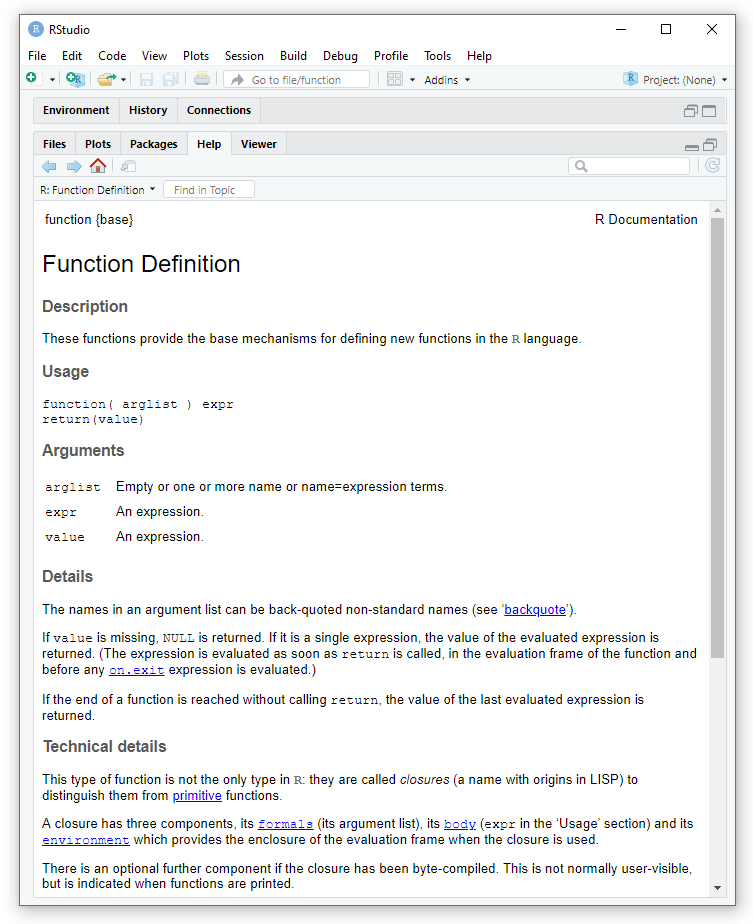# formal style
return(xi + 5);
}

# or replace the as with equal sign
# this will makes the R# code more typescript style:
return(xi + 5);
}

in the formal style of a R# function declaration, the symbol name is the function name, the as part expression shows that the type of target symbol that we declared is a function, and the function closure body is the symbol data instance value.

may be the formal style contains a lot of words to write our R# code, so you also can write a R# function in lambda style:

# syntax sugar borrowed from julia language
const f(x) = x + 5;
# syntax sugar from the original R language
const add5 = function(xi) xi + 5;

Please notice that: all of the R# function that we declared in our script is Vectorized, so we don't needs the extra for loop or while loop in our function in most of time:

const f(x) = x + 5;

f([1,2,3,4,5]);
#      6  7  8  9  10

### 2. lambda function & functional programming

The R# language is also a kind of functional programming language, so using the function as the parameter value of another function in R# is also very easy. By the same example of the sapply function that we learned above, we can demonstrate how we do the functional programming in R# language:

const add5 = function(xi) {
return(xi + 5);
}

sapply([1,2,3,4,5], function(x) {
x + 5;
});

may be it is still too much words to write that shows in the above demo code. so, the lambda function is introduced into R# language, to make the code of functional programming in R# more simple:

sapply([1,2,3,4,5], x -> x + 5);

### 3. pipeline compares the extension function

There is a greate language programming feature in .NET, which is called extension method: by tag the target static function with ExtensionAttribute in VisualBasic.NET language, that we can make the target function call to a style of object instance method liked. with the extension method, we can chaining our function calls in .NET and build a data pipeline.

A pipeline operator is introduced into R# language when compares with the original R language. the pipeline operator will makes all of the R# function can be called in pipelined way naturally. example as:

const add5 = function(x) {
return(x + 5);
}

[1,2,3,4,5]
# we even can pipeline the anonymous function
# in R# language
|> (function(x) {
return(x ^ 2);
})
;

### 4. expression based and statement based

the VisualBasic language is a kind of statement based language, which it means most of the VisualBasic code not produce value to us unless the VB statement expression is a function invoke. unlike the VisualBasic language, the R# programming language is expression based, which means all of the R# code can produce value. Here is an example that it is clearly enough to show the difference between the two language:

Dim x As Double

If test1 Then
x = 1
Else
x = -1
End If

As you can see, in the code that show above, due to the reason of VB code is statement based, so the If block can not produce value, so we needs to assign the value of variable x in two statements. in different, the R# language is expression based, so we can get the result value from such if branch code directly:

const x as double = {
if (test1) {
1;
} else {
-1;
}
}

## Dataset in R# language

there are 4 primitive data type in R# language, and all of the primitive type in R# language is a kind of atomic vector:

R# Primitive VisualBasic.NET Note
num Single, Double Single will be convert to Double
int Short, Integer, Long Short, Integer will be convert to Long
raw Byte value in range [0,255]
chr Char, String The Char and String comes from VisualBasic.NET is unify as character in R# runtime, and the Char is a kind of special string: its nchar value equals to 1
logi Boolean except TRUE and FALSE, the literal of logical value in R# also can be true, false, yes, no
any Object Any kind of .NET object in R# language is also a faked primitive type

based on these primitive type, then we can compose a more complex data type in R# language:

### key-value paired list

the list type in R# language is kind of a Structure liked data type in VisualBasic. the list type is very flexible: you can store any kind of the data in the value slot, but the key name in a list must be character type. You can create a list via list function, example as:

list(a = 1, b = 2, flag = [TRUE, FALSE], c = "Hello world!")
# List of 4
#  $a : int 1 #$ b    : int 2
#  $flag : logical [1:2] TRUE FALSE #$ c    : chr "Hello world!"

Instead of the list function, a more syntax sugar liked language feature was introduce to the R# language: the JSON literal:

# json literal in R# language will also produce a list object
{
a: 1,
b: 2,
flag: [TRUE, FALSE],
c: "Hello world!"
}
# List of 4
#  $a : int 1 #$ b    : int 2
#  $flag : logical [1:2] TRUE FALSE #$ c    : chr "Hello world!"

for reference a slot value in a R# key-value paired list, we can used the $operator if we know the name, and use the [[xxx]] indexer syntax if we don't know the slot name. example as: const x = list(a = 1, b = 2, flag = [TRUE, FALSE], c = "Hello world!"); # TRUE, FALSE x$flag

for(name in names(x)) {
# the code we demonstrate at here is kind of
# reflection liked code in .NET
print(x[[name]]);
}

### dataframe

the dataframe type in R# language is kind of 2D table. Each column in the R# dataframe is a kind of atomic vector data. you can treat the dataframe in R# language as a kind of special key-value paired list object. the data type between the columns in a dataframe could be variational.

Create a dataframe object can be done via the data.frame function:

data.frame(a = 1, b = 2, c = "Hello world!", flag = [TRUE, FALSE]);
#                a         b              c      flag
# ----------------------------------------------------
# <mode> <integer> <integer>       <string> <boolean>
# [1, ]          1         2 "Hello world!"      TRUE
# [2, ]          1         2 "Hello world!"     FALSE

or dataframe can be cast from a list data object via the as.data.frame function:

as.data.frame(list(a = 1, b = 2, c = "Hello world!", flag = [TRUE, FALSE]));
#                a         b              c      flag
# ----------------------------------------------------
# <mode> <integer> <integer>       <string> <boolean>
# [1, ]          1         2 "Hello world!"      TRUE
# [2, ]          1         2 "Hello world!"     FALSE

the difference between the key-value list and the dataframe object is that: the value in a list could be any kind of the data, by the value in a dataframe should be a atomic vector. and there is a more obvious difference about the vector data between the list and dataframe is the vector size: all of the vector size in a list can be variational, but the vector size in each column of the dataframe should be in size of 1 element or n elements where the n elements must equals to the number or rows of the dataframe. Here is an error example about the create a dataframe in different vector size:

data.frame(a = 1, b = [1,2,3], f = [TRUE, FALSE]);
#  Error in <globalEnvironment> -> data.frame
#   1. arguments imply differing number of rows
#   2. a: 1
#   3. b: 3
#   4. f: 2
#
#  R# source: Call "data.frame"("a" <- 1, "b" <- [1, 2, 3], "f" <- [True, False])
#
# base.R#_interop::.data.frame at REnv.dll:line <unknown>
# SMRUCC/R#.global.<globalEnvironment> at <globalEnvironment>:line n/a

based on the the atomic vector, list, and dataframe data types, we have the enough components to create a R# script to solve a specific scientific problem.

### visit any .NET object in R#

Besides the R# vector, list and dataframe, there is another kind of data type in R# language: the native .NET object. Yes, we can interop the R# code with .NET code directly. For visit the data property of a given .NET object instance, the .NET object property reference syntax in PowerShell language is introduced to the R# language, example like there is a Class definition in VisualBasic:

Class metadata
Public Property name As String
Public Property features As Double()
End Class

then we could read the name property value from the class object that we show above:

# this syntax just works for get property
# set property value is not yet supported.
x = new metadata(name = "My Name", features = [1,2,3,4,5]);
[x]::name;

# if the property value is an array of the
# primitive type in R# language, then it will
# be treated as a atomic vector!
[x]::features + 5;
#      6  7  8  9  10

magic!

## Data Visualization in R# language

Except the purpose of create R# language to make our .NET library scriptable, one of the another purpose of create R# language is we can inspect our data in a simple way. For inspect our dataset, we can use the str or print function in R# language. and more exciting, we can plot our data directly in R# environment, for inspect data in a visual way.

Before learn the chartting plot in R#, we should learn how to save the graphics image in R# language. There are two kind of graphics driver in R# environment currently:

• bitmap function for raster image
• wmf function for create window metadata image
• svg function for vector image
• pdf function for use the pdf file as graphics canvas(not working well currently)

as the same as the original R language it does, we should create a graphics device before any data plot, and then write code to plot data. after graphics drawing by code, then we should use the dev.off() function to close the graphics device driver and flush all of the data into target file which is opened by the bitmap or svg graphics driver function.

We can do the graphics plot to a given image file in such R# code pattern, usually:

# for vector image, just simply change the bitmap function to svg function
# svg(file = "/path/to/image.svg");
bimap(file = "/path/to/image.png");
# code for chartting plot
plot(...);
dev.off();

Now we have already known how to create image file in R# language, then we are going to learn how to plot our data in R# environment. There are some primitive chartting plot is already been defined in the R# base environment, which you can used it directly in the R# scripting without install any other third part libraries. Example as scatter plot:# read scatter point data from a given table file
# and then assign to tuple variables
[x, y, cluster] = read.csv("./scatter.csv", row.names = NULL);

# umap scatter with class colors
bitmap(file = "./scatter.png") {
plot(x, y,
class        = cluster,
title        = "UMAP 2D Scatter",
x.lab        = "dimension 1",
y.lab        = "dimension 2",
legend.block = 13,
colorSet     = "paper",
grid.fill    = "transparent",
size         = [2600, 1600]
);
};

Plot your data in R# environment just very simple, yes, we just plot our data! The primitive data plot function in R# environment makes the things simple, but not too much flexible: if we want to do more plot style tweaking, we don't have too much parameters to modify out plot. So here we introduce a graphic chartting library which is written for R# environment: the ggplot package.

### ggplot for R#

the ggplot package is a R language ggplot2 package liked grammar of graphics library for R# language programming. The R# language is another scientific computing language which is designed for .NET runtime, R# is evolved from the R language. There is a famous graphics library called ggplot2 in R language, so keeps the same, there is a graphics library called ggplot was developed for R# language.

By using the ggplot package, then we can do the data chartting in .NET environment in a more convenient and flexible way. example as stat plots in R# via ggplot: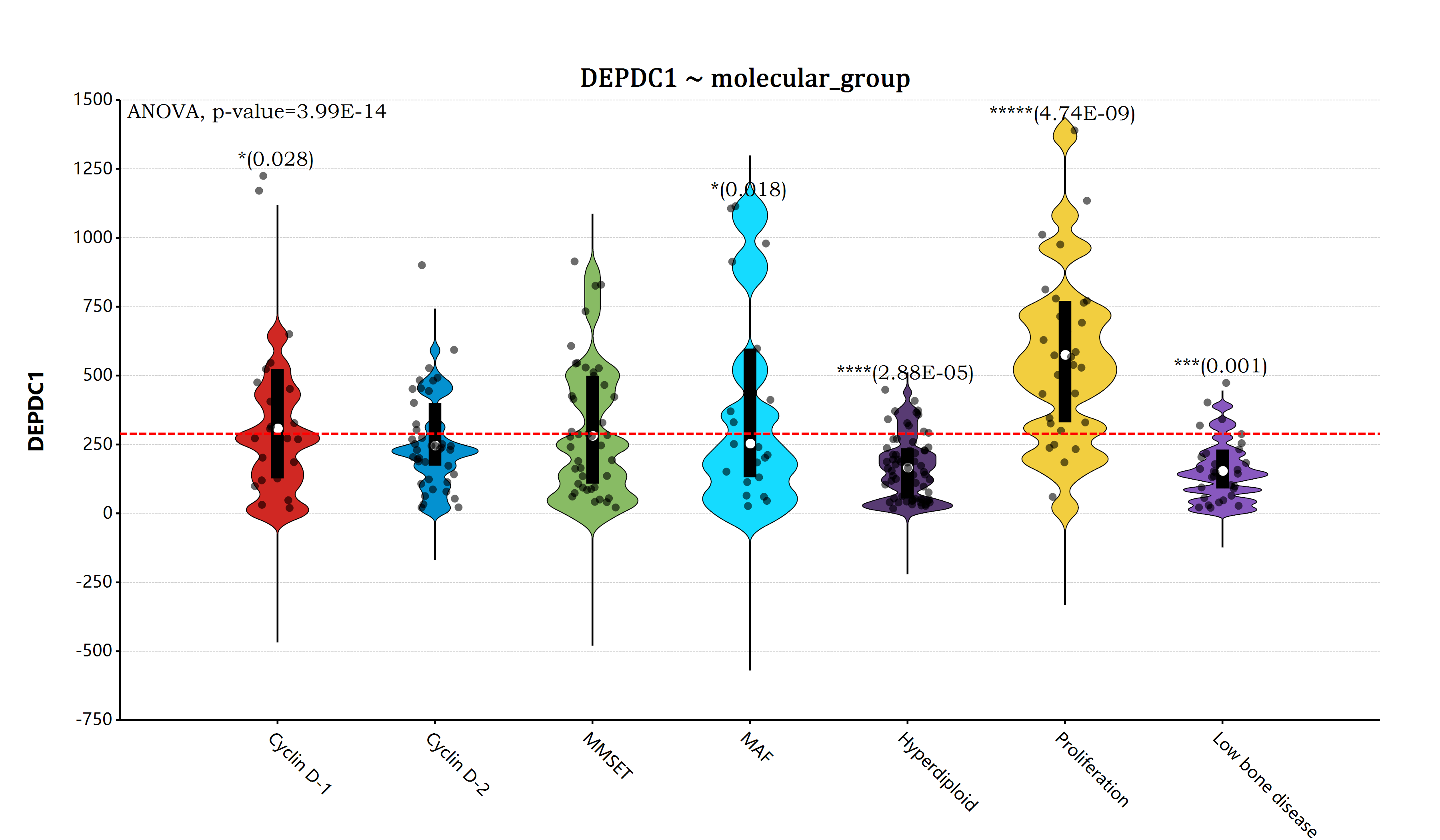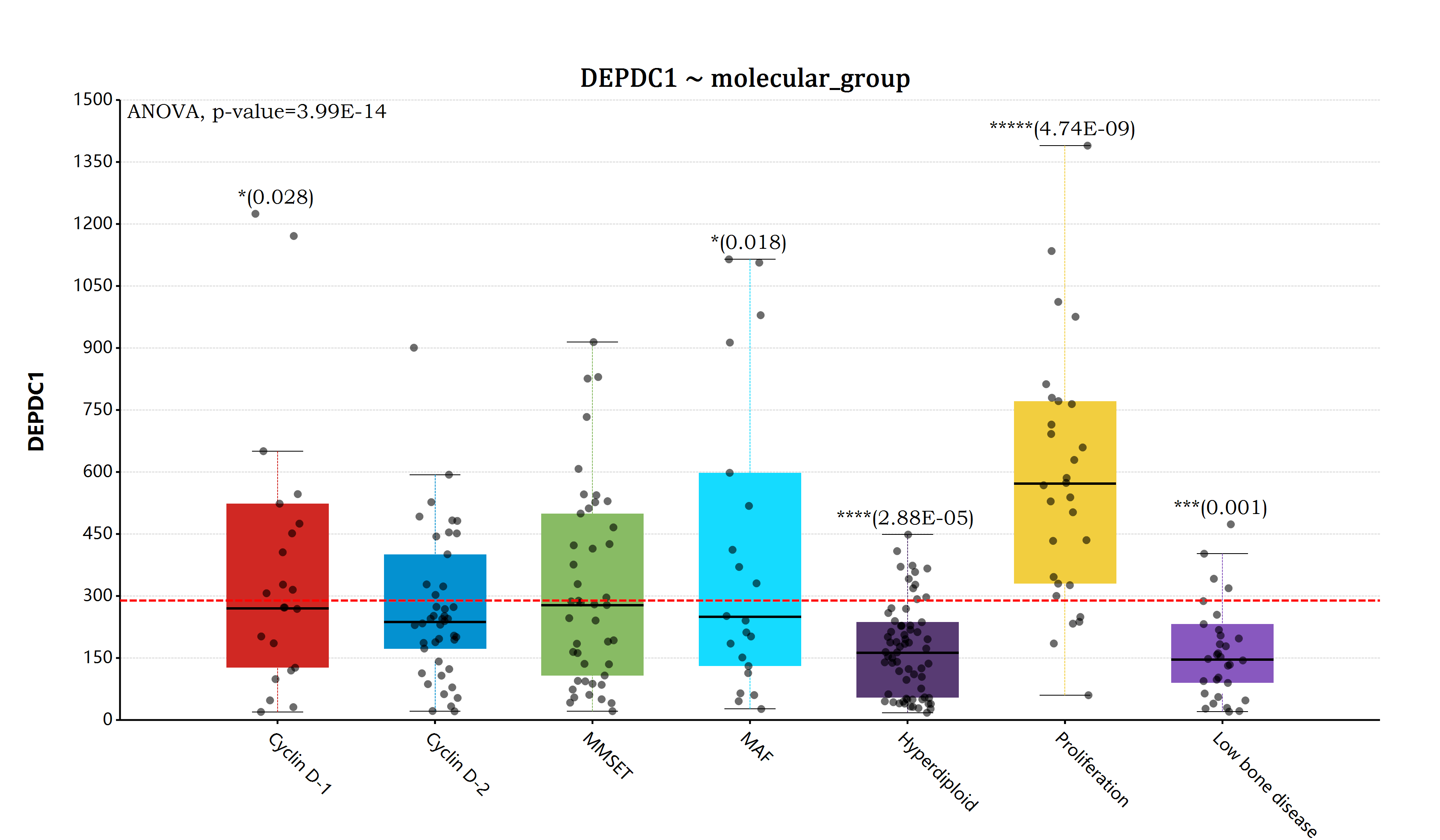ggplot(myeloma, aes(x = "molecular_group", y = "DEPDC1"))
+ geom_boxplot(width = 0.65)
+ geom_jitter(width = 0.3)
# Add horizontal line at base mean
+ geom_hline(yintercept = mean(myeloma\$DEPDC1), linetype="dash", line.width = 6, color = "red")
+ ggtitle("DEPDC1 ~ molecular_group")
+ ylab("DEPDC1")
+ xlab("")
+ scale_y_continuous(labels = "F0")
+ stat_compare_means(method = "anova", label.y = 1600)
# Pairwise comparison against all
+ stat_compare_means(label = "p.signif", method = "t.test", ref.group = ".all.", hide.ns = TRUE)
+ theme(
axis.text.x = element_text(angle = 45),
plot.title  = element_text(family = "Cambria Math", size = 16)
)
;

### ggraph for R#

It is not so easy to make network graph data visualization in .NET environment. The ggplot package for R# is also provides a package module that can be used for the network graph data visualization in a simple way, this package is named ggraph.As we mention above, doing data visualization using the ggplot package in .NET environment is super easy and flexible. we just combine of the ggraph and ggplot, then we can write the elegant code for the network graph data visualization:

ggplot(g, padding = "padding: 50px 300px 50px 50px;")
+ geom_node_convexHull(aes(class = "group"),
alpha        = 0,
stroke.width = 0,
spline       = 0,
scale        = 1.25
)
+ geom_edge_link(color = "black", width = [1,6])
+ geom_node_point(aes(
size  = ggraph::map("degree", [12, 50]),
fill  = ggraph::map("group", "paper"),
shape = ggraph::map("shape", pathway = "circle", metabolite = "Diamond")
)
)
+ geom_node_text(aes(size = ggraph::map("degree", [4, 9]), color = "gray"), iteration = -5)
+ layout_springforce(
stiffness      = 30000,
repulsion      = 100.0,
damping        = 0.9,
iterations     = 10000,
time_step = 0.0001
)
+ theme(legend.text = element_text(
family = "Bookman Old Style",
size = 4
))
;

The ggplot and ggraph R# package is developed inspired by the ggplot2 package for R language, so that many of the function usage can be referenced to the ggplot2 package. Here are the ggplot2 package manual that may be useful for using ggplot chartting function in R# .NET environment.

Latest posts by 谢桂纲 (see all)

### Order by Date Name Attachments

• RFunction_docs • 63 kB • 98 click
31.07.2022

• R-src • 10 kB • 100 click
31.07.2022

• REnv • 169 kB • 96 click
31.07.2022

• scatter • 486 kB • 99 click
31.07.2022

#### No responses yet

1.2.3.查询字符串以["起始，以"]结尾： SELECT * FROM biodeepdb_full.metabolite WHERE INSTR(name, '["') = 1 AND INSTR(name, '"]') = LENGTH(name) - LENGTH('"]') + 1 ORDER…

4.删除mysql表中重复的行数据  delete t1 from metabolite t1 where t1.id in (select t2.id from (select id, name`, formula, hashcode2, row_number() over(partition…

5.